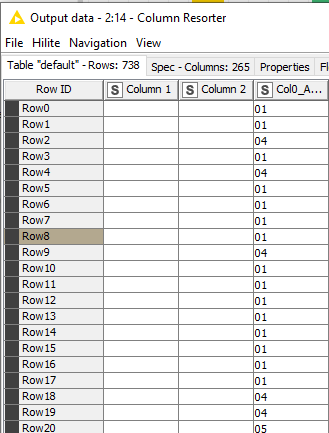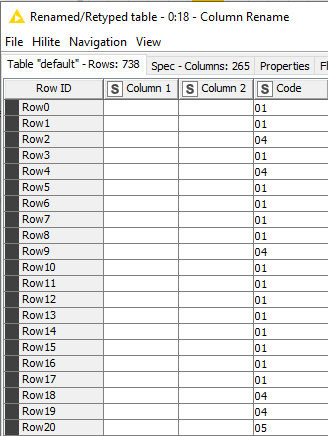# Nested IF Statements

I am brand new to Knime. I am trying to execute a nested if statement on the following table.(thousands of rows long)

Here is the Excel formula =IF(C2=1,IF(C1=1,“YES”,“NO”),IF(C2="",IF(C1=1,“YES”,“NO”),“NO”)).

Any help on which node(s) to use and the proper syntax would be greatly appreciated.

Thanks, TJP6

I think you need the `Rule Engine` node:

There are some examples there which you can hopefully use to get you started - I think what you want is:

``````\$Column 2\$ = 1 AND \$Column 1\$ = 1 => "YES"
\$Column 2\$ = 1  => "NO"
\$Column 2\$ = "" AND \$Column 1\$ = 1 => "YES"
\$Column 2\$ = ""  => "NO"
TRUE => "NO"
``````

Steve

6 Likes

Thanks so much for the reply. I’m going to try to do a better job of explaining what I am looking to do.

Here’s the table I want to manipulate:Here is the Excel formula =IF(C2=1,IF(C1=1,“YES”,“NO”),IF(C2="",IF(C1=1,“YES”,“NO”),“NO”)).

Consequently, I’m trying to define IF(Column “Code” Row 1 =1, IF(Column “Code” Row 0=1, “YES”, “NO”),IF(Column “Code” Row 1="",IF(Column “Code” Row 0=1,“YES”, “NO”),“NO”))

I’m not sure how to assign a column and a row to a formula in the Rule Engine.

Again, I appreciate your help! TJP6

Hi there @TJP6,

welcome to KNIME Community!

Rule Engine node (and pretty much every KNIME node) works row wise. So in your case you need to bring value from previous row one step down. To do that use Lag Column node. Then you should modify syntax provided by @s.roughley in order to simulate Excel formula in KNIME.

Br,
Ivan

1 Like

This topic was automatically closed 182 days after the last reply. New replies are no longer allowed.